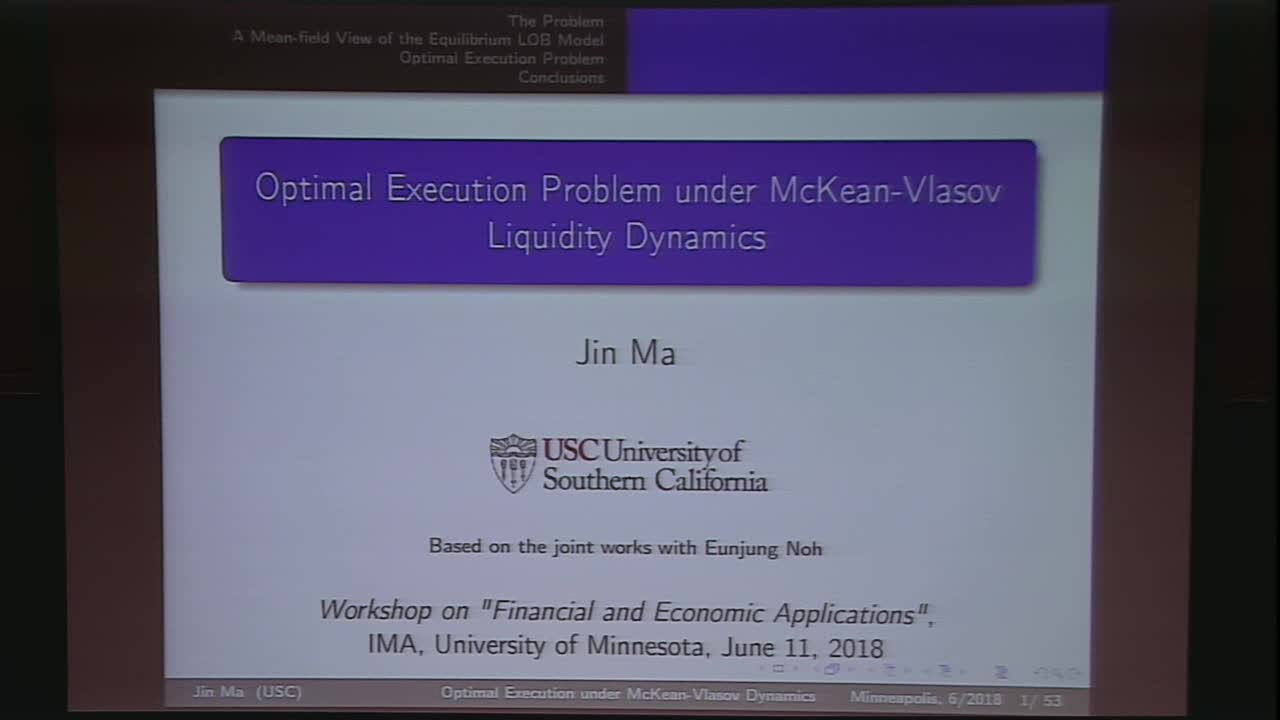## Optimal Execution Problem under McKean-Vlasov Liquidity Dynamics

June 11, 2018
IMA
Presenters: Jin Ma

Watch Video

#### Abstract

In this talk we present some recent new findings regarding optimal execution problems in an order-driven market where the limit order book (LOB) follows the so-called "equilibrium" model. More precisely, we argue that the "equilibrium utility function", which determines both the "shape" and the "frontier" of the LOB endogenously, is in fact the value function of a mean-field type stochastic control problem resulted from a Bertrand-type of game among a large number of sellers. Consequently, the optimal execution problem will be reduced to a sequential game for which two optimal control problems will be involved: one determines the shape of a limit order book (LOB), followed by one that determines the optimal execution strategy. We shall argue that the dynamics of the total liquidity follows a pure-jump, Mckean-Vlasov type SDE with reflection, and is the optimal trajectory of a representing seller in the limiting Bertrand game. The optimal execution problem is then the second optimization problem, which
features a singular type of stochastic control problem with reflected McKean-Vlasov dynamics with jumps. We shall prove the well-posedness of such SDE, verify the Dynamic Programming Principle (DPP) for both optimization problems, and show that both value functions are viscosity solutions of the corresponding HJB equations, which are of the forms of mean-field type integro-partial-differential equation and quasi-variational inequality, respectively.

This talk is based on the joint works with Eunjung Noh.